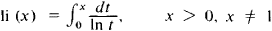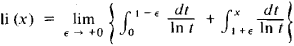# Logarithmic Integral

Also found in: Acronyms, Wikipedia.
The following article is from The Great Soviet Encyclopedia (1979). It might be outdated or ideologically biased.

## Logarithmic Integral

a special function defined by the integralThis integral cannot be expressed in closed form by elementary functions. If x > 1, then the integral is understood in the sense of its principal value:The logarithmic integral was introduced into mathematical analysis by L. Euler in 1768. The logarithmic integral li (x) is connected to the exponential integral Ei (x) by the relation li (x) = Ei (ln x). For large positive x, the function li (x) grows as x/ln x. The logarithmic integral plays an important role in analytic number theory, since the number of primes not exceeding x is approximately equal to li (x).

### REFERENCE

Jahnke, E., F. Emde, and F. Lösch. Spetsial’nye funktsii. Formuly, grafiki, tablitsy. Moscow, 1968. (Translated from German.)